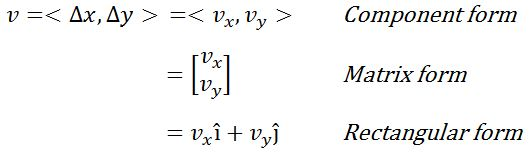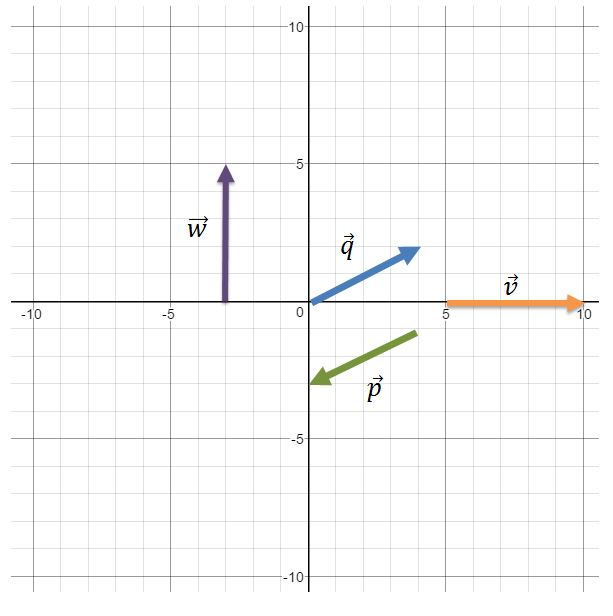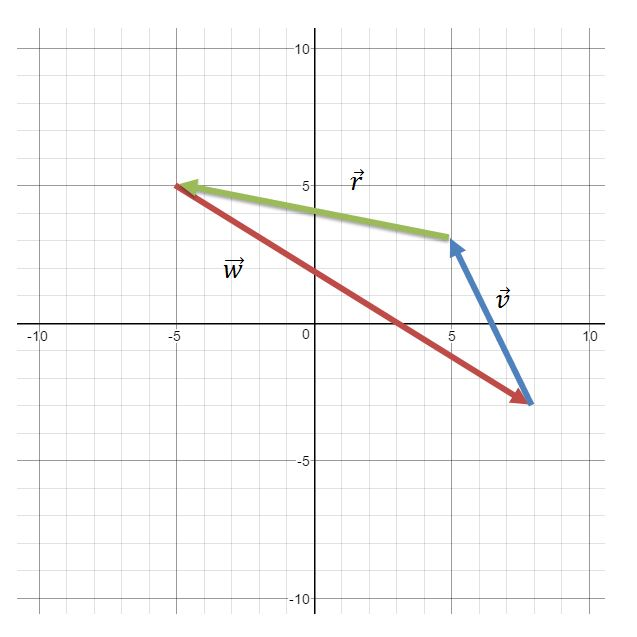# Introduction to vectors

### Introduction to vectors

The notion of vectors can be easily found in our daily surroundings – from airplane navigation to trajectories of balls during a sports game, to movements of a character you maneuver in your video games. In this section, we will learn about the graphical, and mathematical representation of a vector, as well as how to distinguish the differences between a scalar quantity and a vector quantity.

#### Lessons

Vector Quantity: a quantity having (positive) magnitude and (one) direction
Scalar Quantity: a quantity having only magnitude but not direction
Two – Dimensional Vectors:• Introduction

• 1.
Which of the following is/are a vector?
i)
$50$ miles per hour north
ii)
$\sqrt{40 } km/s^2$
iii)
$-43.1^{\circ} C$
iv) $5641\; ft^3$
v)
$32$ Newton at $98^{\circ}$ west of north

• 2.
For the following vectors, express them in component form, matrix form, and rectangular forma)
$\vec{p}$

b)
$\vec{q}$

c)
$\vec{v}$

d)
$\vec{w}$

• 3.
For the following vectors, express them in component form, matrix form, and rectangular forma)
$\vec{v}$

b)
$\vec{w}$

c)
$\vec{r}$